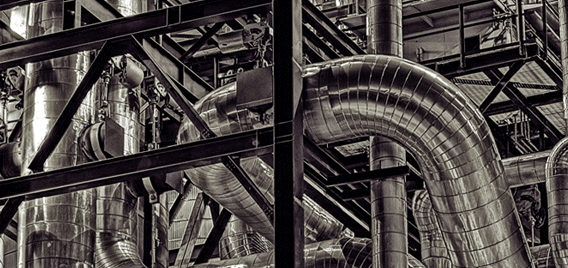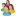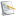### Chemical and Process Engineering Resources## Using Equivalent Lengths of Valves and Fittings

Nov 08 2010 01:20 PM | pleckner in Fluid FlowAn Example

The fluid being pumped is 94% Sulfuric Acid through a 3", Schedule 40, Carbon Steel pipe:

 Mass Flow Rate, lb/hr 63,143 Volumetric Flow Rate, gpm 70 Density, lb/ft3 112.47 S.G. 1.802 Viscosity, cp 10 Temperature, oF 127 Pipe ID, in 3.068 Velocity, ft/s 3.04 Reynold's No 12,998 Darcy Friction Factor, (f) Pipe 0.02985 Pipe Line ?P/100 ft 1.308 Friction Factor at Full Turbulence (ft) 0.018 Straight Pipe, ft 31.5

Â

 Fittings Leq/D1 Leq2, 3 K1, 2 =ft (L/D) Quantity Total Leq Total K 90o Long Radius Elbow 20 5.1 0.36 2 10.23 0.72 Branch Tee 60 15.3 1.08 1 15.34 1.08 Swing Check Valve 50 12.8 0.90 1 12.78 0.90 Plug Valve 18 4.6 0.324 1 4.60 0.324 3" x 1" Reducer4 None5 822.685 57.92 1 822.68 57.92 Total Â Â Â Â 865.63 60.94

Notes:

1. K values and Leq/D are obtained from Reference 1.
2. K values and Leq are given in terms of the larger sized pipe.
3. Leq is calculated using Equation 5 above.
4. The reducer is really an expansion; the pump discharge nozzle is 1" (Schedule 80) but the connecting pipe is 3". In piping terms, there are no expanders, just reducers. It is standard to specify the reducer with the larger size shown first. The K value for the expansion is calculated as a gradual enlargement with a 30o angle.
5. There is no L/D associated with an expansion or contraction. The equivalent length must be back calculated from the K value using Equation 5 above.
 Â Typical Equivalent Length Method K Value Method Straight Pipe ?P, psi Not Applicable 0.412 Total Pipe Equivalent Length ?P, psi 11.734 Not Applicable Valves and Fittings ?P, psi Not Applicable 6.828 Total Pipe ?P, psi 11.734 7.240

The line pressure drop is greater by about 4.5 psi (about 62%) using the typical equivalent length method (adding straight pipe length to the equivalent length of the fittings and valves and using the pipe line fiction factor in Equation 1).

One can argue that if the fluid is water or a hydrocarbon, the pipeline friction factor would be closer to the friction factor at full turbulence and the error would not be so great, if at all significant; and they would be correct. However hydraulic calculations, like all calculations, should be done in a correct and consistent manner. If the engineer gets into the habit of performing hydraulic calculations using fundamentally incorrect equations, he takes the risk of falling into the trap when confronted by a pumping situation as shown above.

Another point to consider is how the engineer treats a reducer when using the typical equivalent length method. As we saw above, the equivalent length of the reducer had to be back-calculated using equation 5. To do this, we had to use ft and K.

Why not use these for the rest of the fittings and apply the calculation correctly in the first place?

### Fluid Flow Articleslidachang

yeal,thank you very much,i got a lot from  this topic.ksb
In Crane Tech Paper 410 (1982 version), Example 4-14 shows a 5"x4" Reducing Elbow which they model as a straight size 5" elbow and a sudden enlargement 4" to 5". In step 4 of Example 4-14, they calculate a pressure increase (negative pressure drop) according to the Bernoulli theorem, because the water slows down as it flows from the smaller diameter to the larger diameter. I was wondering why you didn't include the velocity change in your Sulfuric Acid example since it flows from the 1" pump discharge nozzle through the reducer to the 3" pipe. Since the velocity in the 1" nozzle is 31.22 ft/s, the Bernoulli dP would be -11.72 psi plus the frictional dP of 7.24 psi for a total pressure drop of -4.48 psi which is a pressure increase.I'm a bit confused by the preferred equations to be used. The only equation that produces the 11.73 psi dP for the system described is Equation 2, yet Equations 6 & 7 seem to be preferred.

•Industrial Forum

•Student Forum

•Refining Forum

•Simulation Forum

•Relief Devices Forum

•Tank Venting Forum

•Separation Technology

•Calculations and Tips

•Fluid Flow

•Heat Transfer

•Maintenance and Repair

•Utilities

•Safety and Pressure Relief

•Bulk Solids

•Process and Reactions

•Energy

•Other Topics

•For Students

•Physical Properties

•Archived Articles